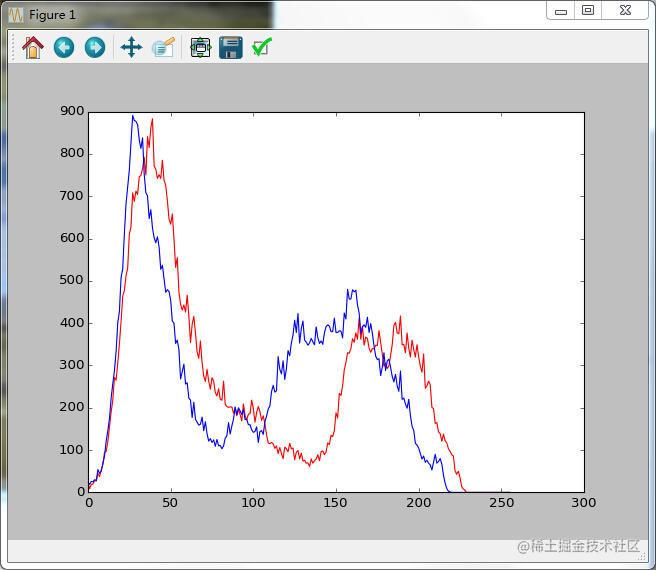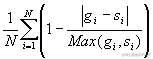# Python+Opencv识别两张相似图片

## 直方图计算法

[图片上传失败...(image-6ca66e-1617780875489)]## 平均哈希法(aHash)

• 1.缩放图片，一般大小为8*8，64个像素值。
• 2.转化为灰度图
• 3.计算平均值：计算进行灰度处理后图片的所有像素点的平均值，直接用numpy中的mean()计算即可。
• 4.比较像素灰度值：遍历灰度图片每一个像素，如果大于平均值记录为1，否则为0.
• 5.得到信息指纹：组合64个bit位，顺序随意保持一致性。

## 感知哈希算法(pHash)

• 缩小图片：32 * 32是一个较好的大小，这样方便DCT计算
• 转化为灰度图
• 计算DCT：利用Opencv中提供的dct()方法，注意输入的图像必须是32位浮点型，所以先利用numpy中的float32进行转换
• 缩小DCT：DCT计算后的矩阵是32 * 32，保留左上角的8 * 8，这些代表的图片的最低频率
• 计算平均值：计算缩小DCT后的所有像素点的平均值。
• 进一步减小DCT：大于平均值记录为1，反之记录为0.
• 得到信息指纹：组合64个信息位，顺序随意保持一致性。

## dHash算法

• 缩小图片：收缩到9*8的大小，以便它有72的像素点
• 转化为灰度图
• 计算差异值：dHash算法工作在相邻像素之间，这样每行9个像素之间产生了8个不同的差异，一共8行，则产生了64个差异值
• 获得指纹：如果左边的像素比右边的更亮，则记录为1，否则为0.
• 最后比对两张图片的指纹，获得汉明距离即可。

``````# -*- coding: utf-8 -*-
# 利用python实现多种方法来实现图像识别

import cv2
import numpy as np
from matplotlib import pyplot as plt

# 最简单的以灰度直方图作为相似比较的实现
def classify_gray_hist(image1,image2,size = (256,256)):
# 先计算直方图
# 几个参数必须用方括号括起来
# 这里直接用灰度图计算直方图，所以是使用第一个通道，
# 也可以进行通道分离后，得到多个通道的直方图
# bins 取为16
image1 = cv2.resize(image1,size)
image2 = cv2.resize(image2,size)
hist1 = cv2.calcHist([image1],,None,,[0.0,255.0])
hist2 = cv2.calcHist([image2],,None,,[0.0,255.0])
# 可以比较下直方图
plt.plot(range(256),hist1,'r')
plt.plot(range(256),hist2,'b')
plt.show()
# 计算直方图的重合度
degree = 0
for i in range(len(hist1)):
if hist1[i] != hist2[i]:
degree = degree + (1 - abs(hist1[i]-hist2[i])/max(hist1[i],hist2[i]))
else:
degree = degree + 1
degree = degree/len(hist1)
return degree

# 计算单通道的直方图的相似值
def calculate(image1,image2):
hist1 = cv2.calcHist([image1],,None,,[0.0,255.0])
hist2 = cv2.calcHist([image2],,None,,[0.0,255.0])
# 计算直方图的重合度
degree = 0
for i in range(len(hist1)):
if hist1[i] != hist2[i]:
degree = degree + (1 - abs(hist1[i]-hist2[i])/max(hist1[i],hist2[i]))
else:
degree = degree + 1
degree = degree/len(hist1)
return degree

# 通过得到每个通道的直方图来计算相似度
def classify_hist_with_split(image1,image2,size = (256,256)):
# 将图像resize后，分离为三个通道，再计算每个通道的相似值
image1 = cv2.resize(image1,size)
image2 = cv2.resize(image2,size)
sub_image1 = cv2.split(image1)
sub_image2 = cv2.split(image2)
sub_data = 0
for im1,im2 in zip(sub_image1,sub_image2):
sub_data += calculate(im1,im2)
sub_data = sub_data/3
return sub_data

# 平均哈希算法计算
def classify_aHash(image1,image2):
image1 = cv2.resize(image1,(8,8))
image2 = cv2.resize(image2,(8,8))
gray1 = cv2.cvtColor(image1,cv2.COLOR_BGR2GRAY)
gray2 = cv2.cvtColor(image2,cv2.COLOR_BGR2GRAY)
hash1 = getHash(gray1)
hash2 = getHash(gray2)
return Hamming_distance(hash1,hash2)

def classify_pHash(image1,image2):
image1 = cv2.resize(image1,(32,32))
image2 = cv2.resize(image2,(32,32))
gray1 = cv2.cvtColor(image1,cv2.COLOR_BGR2GRAY)
gray2 = cv2.cvtColor(image2,cv2.COLOR_BGR2GRAY)
# 将灰度图转为浮点型，再进行dct变换
dct1 = cv2.dct(np.float32(gray1))
dct2 = cv2.dct(np.float32(gray2))
# 取左上角的8*8，这些代表图片的最低频率
# 这个操作等价于c++中利用opencv实现的掩码操作
# 在python中进行掩码操作，可以直接这样取出图像矩阵的某一部分
dct1_roi = dct1[0:8,0:8]
dct2_roi = dct2[0:8,0:8]
hash1 = getHash(dct1_roi)
hash2 = getHash(dct2_roi)
return Hamming_distance(hash1,hash2)

# 输入灰度图，返回hash
def getHash(image):
avreage = np.mean(image)
hash = []
for i in range(image.shape):
for j in range(image.shape):
if image[i,j] > avreage:
hash.append(1)
else:
hash.append(0)
return hash

# 计算汉明距离
def Hamming_distance(hash1,hash2):
num = 0
for index in range(len(hash1)):
if hash1[index] != hash2[index]:
num += 1
return num

if __name__ == '__main__':
cv2.imshow('img1',img1)
cv2.imshow('img2',img2)
degree = classify_gray_hist(img1,img2)
#degree = classify_hist_with_split(img1,img2)
#degree = classify_aHash(img1,img2)
#degree = classify_pHash(img1,img2)
print degree
cv2.waitKey(0)

## 资源传送门

• 关注【==做一个柔情的程序猿==】公众号

• 在【==做一个柔情的程序猿==】公众号后台回复 【python资料】【2020秋招】 即可获取相应的惊喜哦！

• 自己搭建的博客地址：梦魇回生的博客

## 「❤️ 感谢大家」

• 点赞支持下吧，让更多的人也能看到这篇内容（收藏不点赞，都是耍流氓 -_-）
• 欢迎在留言区与我分享你的想法，也欢迎你在留言区记录你的思考过程